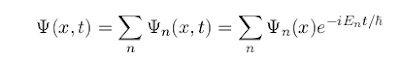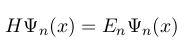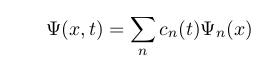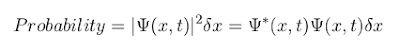Solved Problems in Physics, Physical Chemistry, and Computer Simulation Projects for College and Graduate Students.

# Questions and Solutions in Fundamental Principles of Quantum Mechanics

### Questions:

(a) In what sense is quantum mechanics fundamentally in-deterministic? Give two physical examples of such indeterminacy in real systems.

(b) Describe the role of the Heisenberg uncertainty principle in undermining the Newtonian description of the state of a system.

(c) Outline the role of the wave function in describing the state of an isolated system in one-dimensional wave mechanics. Is the description given by the wave function as complete as can be? Discuss whether the indeterminacy of quantum mechanics arises from our lack of knowledge of the time-evolution of the wave function in between measurements (as described by Schrodinger’s equation), or whether it has another origin.

(d) Describe the role of eigenvalue equations in determining which energy values are possible in a bound system. Are there any situations in which quantum mechanics unambiguously predicts the result of an energy measurement with certainty? How does the collapse of the wave function influence the result of an energy measurement taken immediately after another energy measurement?

(e) Explain the roles of the overlap and coefficient rules in predicting the probability of obtaining a given value when an energy measurement is made in a given state.

(f) Explain the role of Born’s rule in predicting the probability of finding a particle within a given interval? How does the collapse of the wave function influence the result of a position measurement taken immediately after another position measurement?

(g) Finally give a brief summary of the types of prediction that are possible in quantum mechanics. Comment on the extent to which these predictions can (in principle) be compared with experiment by, for example, making repeated measurements.

### Solution:

(a) Quantum mechanics is irreducibly in-deterministic and some things cannot be predicted, even in principle. For instance (i) when a particular radioactive nucleus will decay, remains a matter of pure chance, and (ii) the emission of photons by atoms is also inherently in-deterministic.

(b) In Newtonian mechanics, state of a system can be described by position and momentum of its particles, where all of the particles have accurate values of momentum and position. But according to the Heisenberg uncertainty principle, it is impossible to know both the position and momentum of a particle with perfect accuracy. In fact, the more we know about the position of a particle, the less we can know about its momentum. So according to the quantum mechanics, we cannot describe the state of a system by position and momentum of its particles.

(c) State of an isolated system in one dimension, can be described by the sum of its stationary-state eigen-functions,where En is the energy eigenvalue corresponding to the eigen-function of Ψn(x).

Probability distribution of energy of an isolated system remains constant in time.

Yes, it is as complete as can be. The set of stationary-state eigen-functions, Ψn(x, t), is complete, i.e. the state of the system is completely specified by its wave function which can be expanded in terms of eigen-functions.
It has another origin. Schrodinger equation predicts the time-evoloution of the wave function ( which is a linear superposition of eigen-functions). But any measurement will collapse the wave function to a definite eigen-state.
This is a sudden and abrupt change that is not described by Schrodinger equation. So Schrodinger equation can predict the time evolution of wave function between two measurements, and this is the measurement itself which has an unpredictable effect on the wave function.

(d) Eigenvalues of the Hamiltonian of a bound system, are the possible values of the energy of the system,Yes, if a system is described by a specific eigen-state (e.g. Ψn(x, t)) of its stationary-state wave function, by measuring its energy, we will be able to find eigenvalue, En corresponding to this eigen-state.

The first measurement will cause the collapse of the wave function to one of eigen-functions, e.g. Ψn(x, t), with corresponding eigenvalue of energy, En . By performing second measurement of energy, immediately after the first measurement, the result will be En (that is obtained in the first measurement). So the result of an energy measurement taken immediately after another energy measurement, is absolutely predictable, due to the collapse of the wave function.

(e) By measuring the energy of a system, we can obtain one of its energy eigenvalues, En . We can use the overlap or coefficient rules and find the probability of obtaining a given value of energy.

Overlap rule: the probability of obtaining eigenvalue of energy, En , can be found from the overlap integral of the wave function of the system, Ψ(x, t), and the energy eigen-function, Ψn(x), corresponding to the energy eigenvalue En ,where both the wave function and eigen-function are normalized.
Coefficient rule: if the wave function of a system, Ψ(x, t) is the linear superposition of the energy eigen-functions,the probability of obtaining energy eigenvalue En , by energy measurement, is,where cn(t) is the coefficient of the energy eigen function Ψn(x) in the wave function at the time of measurement, and both the wave function and eigen-function are normalized.

(f) According to the Born’s rule the probability of finding a particle in a small region is proportional to the square of the modulus of the wave function in that region. For instance, in one-dimension, the probability of finding a particle in a small interval of length δx, centered on x, is,where Ψ(x, t) is the normalized wave function of the particle. |Ψ(x, t)|2 is the probability density.

So the probability of finding the particle within a given interval, is equal to the integration of probability over the given interval. For instance in one dimension the probability of finding the particle in an space interval between x=a and x=b, can be found as,Position measurement causes the collapse of the wave function of the particle, and just after the measurement the particle settles in one of its eigen-states with a a specific eigenvalue, that is the position of the particle just after the measurement. By performing another position measurement immediately after the first measurement, the result will be the same as the result obtained from the first measurement (a specific eigenvalue of position). So the second measurement, immediately after the first one, is absolutely predictable.

(g)
-Time evolution of the wave function of a system, which contains all of the information about the system, is predictable by Schrodinger equation, and by using Born’s rule, probability distribution of its physical parameters (e.g. position, momentum, energy,...) are predictable. So, we can predict all of the possible values of the physical parameters of the system, and the probability of all of these possible values.

-In quantum mechanics, the average behavior of a system containing large number of identical particles, is absolutely predictable ( although the behavior of any individual particle in the system is not predictable).

-A system that is in a stationary-state is also absolutely predictable. For instance just after a measurement is done, due to the collapse of the wave function of the system, the system is in one of its eigen-states and the corresponding eigenvalue would be the outcome of any immediate measurement; so we can predict the result of the same measurement at that time.

We can see the predictable phenomena in practice, such as diffraction pattern produced by large number of electrons, the fact that the half-lives measured for any two large collections of identical nuclei will always be the same wherever and whenever they are measured, that copper will always be reddish in colour and the energy levels of hydrogen will always be the same. Also by performing repeated measurements of a physical parameter of a system, i.e. a measurement proceeded immediately after the first measurement, we can obtain a completely predictable result (the outcome of the first measurement corresponding to the state of the system just after the first measurement).